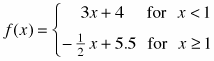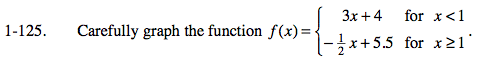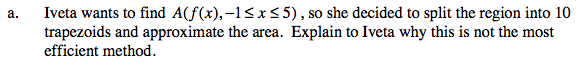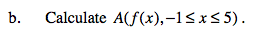### Home > CALC > Chapter 1 > Lesson 1.3.3 > Problem1-125

1-125.
1. Carefully graph the function. 1-125 eTool (Desmos) Homework Help ✎

1. Iveta wants to find A(f(x), −1 ≤ x ≤ 5), so she decided to split the region into 10 trapezoids and approximate the area. Explain to Iveta why this is not the most efficient method.

2. Calculate A(f(x), −1 ≤ x ≤ 5).Can you find a way to calculate the area accurately with less than 10 trapezoids?

Because the function is composed of two linear pieces, the exact area can be computed using two trapezoids.Use the eTool below to visualize this problem.
Click the link at right for the full version of the eTool: Calc 1-125 HW eTool